# DE102016202501A1 - Method for determining a calibration current pulse - Google Patents

Method for determining a calibration current pulse

## Info

Publication number
DE102016202501A1
DE102016202501A1 DE102016202501.5A DE102016202501A DE102016202501A1 DE 102016202501 A1 DE102016202501 A1 DE 102016202501A1 DE 102016202501 A DE102016202501 A DE 102016202501A DE 102016202501 A1 DE102016202501 A1 DE 102016202501A1
Authority
DE
Germany
Prior art keywords
measuring resistor
connection point
resistor
measuring
path
Prior art date
Legal status (The legal status is an assumption and is not a legal conclusion. Google has not performed a legal analysis and makes no representation as to the accuracy of the status listed.)
Pending
Application number
DE102016202501.5A
Other languages
German (de)
Inventor
Ralf Schröppel
Wolfgang Weigert
Current Assignee (The listed assignees may be inaccurate. Google has not performed a legal analysis and makes no representation or warranty as to the accuracy of the list.)
Continental Automotive GmbH
Original Assignee
Continental Automotive GmbH
Priority date (The priority date is an assumption and is not a legal conclusion. Google has not performed a legal analysis and makes no representation as to the accuracy of the date listed.)
Filing date
Publication date
Application filed by Continental Automotive GmbH filed Critical Continental Automotive GmbH
Priority to DE102016202501.5A priority Critical patent/DE102016202501A1/en
Publication of DE102016202501A1 publication Critical patent/DE102016202501A1/en
Pending legal-status Critical Current

• 244000171263 Ribes grossularia Species 0.000 title claims description 102
• 238000004364 calculation methods Methods 0.000 abstract description 6
• 238000000034 methods Methods 0.000 description 5
• 239000000463 materials Substances 0.000 description 4
• 230000001419 dependent Effects 0.000 description 3
• 239000000203 mixtures Substances 0.000 description 3
• 230000004044 response Effects 0.000 description 3
• 238000004088 simulation Methods 0.000 description 3
• 230000032683 aging Effects 0.000 description 2
• 230000000875 corresponding Effects 0.000 description 2
• 238000000605 extraction Methods 0.000 description 2
• 230000000051 modifying Effects 0.000 description 2
• 238000003860 storage Methods 0.000 description 2
• 241001236653 Lavinia exilicauda Species 0.000 description 1
• 229910000896 Manganin Inorganic materials 0.000 description 1
• 229910000914 Mn alloys Inorganic materials 0.000 description 1
• 281000009227 Power Balance companies 0.000 description 1
• 229910045601 alloys Inorganic materials 0.000 description 1
• 239000000956 alloys Substances 0.000 description 1
• 230000006399 behavior Effects 0.000 description 1
• 238000004422 calculation algorithm Methods 0.000 description 1
• 238000010276 construction Methods 0.000 description 1
• 230000002596 correlated Effects 0.000 description 1
• 230000018109 developmental process Effects 0.000 description 1
• 238000010586 diagrams Methods 0.000 description 1
• 238000009826 distribution Methods 0.000 description 1
• 230000000694 effects Effects 0.000 description 1
• 238000005265 energy consumption Methods 0.000 description 1
• 238000005516 engineering processes Methods 0.000 description 1
• 238000004519 manufacturing process Methods 0.000 description 1
• 238000001228 spectrum Methods 0.000 description 1
• 239000007858 starting materials Substances 0.000 description 1
• 230000001629 suppression Effects 0.000 description 1
• 230000036962 time dependent Effects 0.000 description 1

## Images

•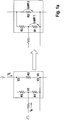••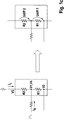•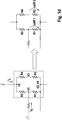•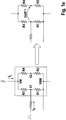•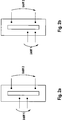•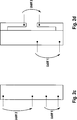•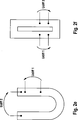••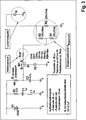•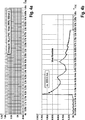•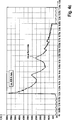•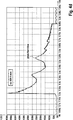•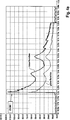•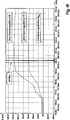••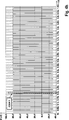•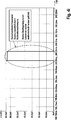•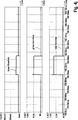•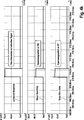## Classifications

• GPHYSICS
• G01MEASURING; TESTING
• G01RMEASURING ELECTRIC VARIABLES; MEASURING MAGNETIC VARIABLES
• G01R35/00Testing or calibrating of apparatus covered by the preceding groups
• GPHYSICS
• G01MEASURING; TESTING
• G01RMEASURING ELECTRIC VARIABLES; MEASURING MAGNETIC VARIABLES
• G01R1/00Details of instruments or arrangements of the types included in groups G01R5/00 - G01R13/00 and G01R31/00
• G01R1/20Modifications of basic electric elements for use in electric measuring instruments; Structural combinations of such elements with such instruments
• G01R1/203Resistors used for electric measuring, e.g. decade resistors standards, resistors for comparators, series resistors, shunts
• GPHYSICS
• G01MEASURING; TESTING
• G01RMEASURING ELECTRIC VARIABLES; MEASURING MAGNETIC VARIABLES
• G01R19/00Arrangements for measuring currents or voltages or for indicating presence or sign thereof
• G01R19/0092Arrangements for measuring currents or voltages or for indicating presence or sign thereof measuring current only
• GPHYSICS
• G01MEASURING; TESTING
• G01RMEASURING ELECTRIC VARIABLES; MEASURING MAGNETIC VARIABLES
• G01R19/00Arrangements for measuring currents or voltages or for indicating presence or sign thereof
• G01R19/10Measuring sum, difference or ratio

## Abstract

The invention relates to a method for determining a Kalibrierstrompulses when superimposed by a Nutzstrompuls, the Kalibrierstrompuls is determined by calculation over several measuring resistances of a measuring resistor group.

## Description

• The invention relates to a method for determining a calibration current pulse.
• In automobiles, a battery state detection is increasingly performed to reliably calculate the performance of the battery. A so-called Intelligent Battery Sensor (IBS) is typically used for the detection of the battery measured variables.
• A continuous measurement of the battery state is advantageous in order to optimize the power balance of the motor vehicle and thus make a significant contribution to reducing consumption and CO 2 savings. In addition to the short-term power output during engine starts, innovations such as start-stop operation and recuperation are added in modern vehicles, which lead to increased energy consumption and stress the battery in addition to the already known power outputs.
• A detection of the battery measured quantities current, voltage and temperature should typically be very accurate, dynamic and time-synchronized in order to be able to determine the battery condition, the performance of the battery and its degree of aging, for example by means of an algorithm.
• The measurement of the battery measured variable current today is usually carried out with the aid of a high-quality and precise measuring resistor, also called a measuring shunt, which typically has a drift of its resistance value of less than 1% of the measured value over its service life of, for example, 15 years. However, this measuring resistor is usually very costly with high accuracy and low temperature response. By way of example, a copper-nickel-manganese alloy, in particular an alloy known as manganin, can be used for this purpose.
• In order to be able to use more cost-effective resistance materials or measuring resistors with a higher inaccuracy in the resistance value and temperature response in the future, it is possible to carry out a continuous calibration of the measuring system during operation in order to compensate for the inaccuracies. Such a calibration should advantageously be carried out continuously during the entire service life of the battery sensor when installed in the vehicle.
• This can be done, for example, with a continuous recurring calibration current pulse or calibration current pulse. This calibration current pulse can be generated on the battery sensor board. For example, it generates voltage drops across a reference resistor as well as the measuring resistor across all temperature and load current ranges, which can then be measured and correlated continuously. Thus, a continuous comparison with a reference resistor is possible.
• It has proven to be problematic in continuous calibration methods that the calibration current pulse and the load current are added together and can only be separated from one another again with difficulty, which leads to great problems in the evaluation of the measured data.
• It is therefore an object of the invention to provide a method for determining a calibration current pulse which is improved in this regard.
• This is achieved according to the invention by a method according to claim 1. Advantageous embodiments can be taken, for example, the dependent claims. The content of the claims is made by express reference to the content of the description.
• The invention relates to a method for determining a calibration current pulse on a measuring resistor group. The measuring resistor group has a number of measuring resistors, which are connected at respective connection points with each other or with other components.
• The transition from a measuring resistor to a measuring resistor group enables a special procedure, which is part of the method according to the invention.
• It should be understood that the connection points can be configured in many ways, for example as electrical contacts between physically delimitable resistors. However, it may also be merely specific points or locations on resistance materials which delimit parts of these resistance materials from each other.
• The method comprises the following steps:
• Passing a load current through a first measuring resistor of the measuring resistor group and through a second measuring resistor of the measuring resistor group, simultaneously
• - Passing a calibration current through at least the first measuring resistor, simultaneously
• - Measuring a first voltage pulse between a first connection point and a second connection point, wherein the first connection point is directly connected to the first sense resistor, and
• - Calculating the Kalibrierstrompulses based at least on the first voltage pulse.
• Due to the special procedure, it is possible to obtain a conclusion about the calibration current based on the first voltage pulse, which can be separated from the load current. Possible specific embodiments are described below in the application.
• In particular, the load current is a current which flows from the battery to the chassis or vice versa, for example because consumers in the vehicle have a corresponding power requirement. This may be, for example, starter or headlights.
• A battery may be, for example, a typical car battery, which may be designed in particular as an accumulator. However, the method is also applicable to other batteries or accumulators or general electricity storage or power generator.
• According to one embodiment, it is provided that the second connection point is connected directly to the first measuring resistor, so that the first voltage pulse is measured exactly above the first measuring resistor.
• The method according to an embodiment further comprises, at the same time as the step of measuring the first voltage pulse, the following step:
• - Measuring a second voltage pulse between a third connection point and a fourth connection point, wherein the third connection point is directly connected to the second measuring resistor, and
• - The calibration current pulse is also calculated based on the second voltage pulse.
• By measuring the second voltage pulse, different solutions can be used to extract the calibration current pulse from the superposition with the load current. Exemplary procedures will be described below.
• According to one embodiment, the fourth connection point is directly connected to the second measurement resistor, so that the second voltage pulse is measured exactly above the second measurement resistor.
• According to one embodiment, the second connection point is directly connected to the second measuring resistor.
• Advantageously, the calibration current pulse is also calculated based on a resistance value of the first measuring resistor and / or a resistance value of the second measuring resistor and / or respective resistance values of further measuring resistors of the measuring resistor group. It is possible to fall back on all combinations of the named values, that is, for example, with one of these values, with any two of these values, or with three of these values. In particular, ratios of resistances can be taken into account, as will be explained in more detail below.
• According to a preferred embodiment, the calibration current is conducted serially to the first sense resistor through a reference resistor, and a voltage drop is measured across the reference resistance. This allows accurate determination of the current strength of the calibration current.
• According to one embodiment, the load current is split into a first path and a second path parallel thereto. Preferably, it is divided equally. This corresponds to a possible procedure for separating the load current from the calibration current.
• Hereinafter, certain specific embodiments will be described, which the inventors have recognized as advantageous, but not as sole embodiments for carrying out the method. It should be noted that the voltage pulses are typically measured in accordance with the above information regarding the connection points.
• According to one embodiment, it is provided
• That the first path has the first measuring resistor and, in series, a third measuring resistor,
• - That the first measuring resistor and the third measuring resistor are connected to each other at the first connection point,
• That the second path has the second measuring resistor,
• - That the third measuring resistor and the second measuring resistor are connected to each other at the third connection point, and
• - That the first measuring resistor and the second measuring resistor are connected to each other at the second connection point, which simultaneously forms the fourth connection point.
• According to one embodiment, it is provided
• The first path has the first measuring resistor, a third measuring resistor in series therewith, and a fourth measuring resistor in series therewith,
• That the first measuring resistor and the third measuring resistor are connected to one another at the first connection point,
• - That the first measuring resistor and the fourth measuring resistor are connected to each other at the second connection point,
• that the second path has the second measuring resistor,
• - That the third measuring resistor and the second measuring resistor are connected to each other at the third connection point, and
• - That the fourth measuring resistor and the second measuring resistor are connected to each other at the fourth connection point.
• According to one embodiment, it is provided
• That the first path has the first measuring resistor and, in series, a third measuring resistor,
• - That the first measuring resistor and the third measuring resistor are connected to each other at the first connection point,
• - That the second path has the second measuring resistor and serially to a fourth measuring resistor,
• - That the second measuring resistor and the fourth measuring resistor are connected to each other at the third connection point,
• - That the third measuring resistor and the fourth measuring resistor are connected to one another at a fifth connection point, and
• - That the first measuring resistor and the second measuring resistor are connected to each other at the second connection point, which simultaneously forms the fourth connection point.
• According to one embodiment, it is provided
• That the first path has the first measuring resistor and, in series, a third measuring resistor,
• - That the second path has the second measuring resistor and serially to a fourth measuring resistor,
• - That the first measuring resistor and the third measuring resistor are connected to each other at the first connection point,
• - That the second measuring resistor and the fourth measuring resistor are connected to each other at the second connection point,
• - That the third measuring resistor and the fourth measuring resistor are connected to each other at a further connection point, and
• - That the first measuring resistance and the second measuring resistor are connected to each other at yet another connection point.
• According to one embodiment, it is provided
• - That the first measuring resistor and the second measuring resistor are connected in series with each other,
• That the first measuring resistor and the second measuring resistor are connected to one another at the first connecting point, which simultaneously forms the fourth connecting point,
• - That the second connection point is a first connection point opposite pole of the first sense resistor, and
• - That the third connection point is a fourth connection point opposite pole of the second measuring resistor.
• All of these embodiments allow an advantageous determination of the Kalibrierstrompulses. Details will be discussed below with reference to the accompanying drawings.
• According to a preferred embodiment, the calibration current is introduced into the measuring resistor group at the first connection point. This can be particularly advantageously combined with the embodiments described above.
• According to a development, the measuring resistors of the measuring resistor group are designed as subregions of a planar resistor. This allows easy production of the resistors. Exemplary embodiments are described below with reference to the accompanying drawings. It should be understood, however, that any other embodiments of resistors come into consideration, such as discrete resistors.
• The invention further relates to a battery sensor which is configured to carry out a method according to the invention. In particular, the battery sensor may comprise processor means and memory means, wherein the memory means comprise program code, in the execution of which the processor means execute a method according to the invention or behave accordingly. Additionally, the battery sensor may include a sensing resistor assembly configured as described with respect to the method. All embodiments described with reference to the method apply accordingly as possible device embodiments of a battery sensor. With regard to the method can be used on all described versions and variants.
• The invention further relates to a non-transitory computer-readable storage medium containing program code, in the execution of which a processor executes a method according to the invention. With regard to the method can be used on all described versions and variants.
• Generally speaking, it is a possible basic idea of the continuous calibration, in the installed state of a sensor in the vehicle to generate a calibration current or calibration current, which is then directed so that this voltage drop at a high-precision resistor or reference shunt as well as the measuring resistor or Measurement shunt generated. This voltage drop can thus be continuously differentially measured and the two voltages can be set in proportion.
• If this happens for all known load cases and temperature ranges, then the poorer specific properties of the manganese-free measuring shunt material can be compensated.
• Based on this, the reference resistance should have only minimal aging behavior and temperature response. This is favored because it is only slightly thermally loaded and only small currents flow.
• The problem and difficulty with this method of continuous calibration by means of calibration current via a reference resistor, however, is that both the calibration current and the load current flow via the measuring shunt to be calibrated. These two streams add up and a mixed stream is created. The resulting mixed stream contains portions of both signals that are difficult to separate from each other.
• This is where the invention starts. The load current is first divided over the measuring resistor and the calibration current is fed into the measuring shunt in such a way that it flows in different sizes over the divided measuring resistor. By a simple subtraction of the partial load currents, for example, the calibration current pulse can again be extracted from the load current.
• This method provides a simple and inexpensive way to separate the calibration current signal from the load current. Below, an example of this method is calculated and associated simulation results are displayed.
• The method according to the invention may, for example, allow the generated calibration current to be separated from the load current in such a way that the actual calibration current signal can be used for the further calculation with almost no superpositions. It also provides a simple, fast and cost effective solution to extract the relevant voltage drops from the mixed stream.
• The method of this signal extraction is based, for example, at least in some embodiments, on the method of parallel measurement at the measuring shunt, with simultaneous identical load current signals. The trick may be, for example, that the load current is distributed in equal parts and the calibration current is distributed unevenly over the partial resistors. Subtracting the partial load currents, a part of the calibration current pulse remains.
• In detail, for example, the smaller resistance element can be traversed by a large proportion of the calibration current pulse plus load current, and the larger resistance element can be traversed by a smaller proportion of the calibration current pulse plus an equal proportion of load current.
• Further features and advantages will be apparent to those skilled in the embodiments described below with reference to the accompanying drawings. Showing:
• 1a to 1e : Exemplary embodiments and circuits of a measuring resistor arrangement for carrying out the method according to the invention,
• 2a to 2g : Exemplary embodiments of a measuring resistor arrangement,
• 3 : A block diagram, which serves to illustrate the method, and
• 4a to 4k : Graphs illustrating the process and a simulation.
• The 1a to 1e show exemplary embodiments of a measuring resistor group, which has up to four measuring resistors. Basically, a first measuring resistor with R1, a second measuring resistor with R2, a third measuring resistor with R3 and a fourth measuring resistor with R4 are called.
• The measuring resistors are connected at respective connection points with each other and with external components. The connection points are designated by the reference symbols V1 for a first connection point, V2 for a second connection point, V3 for a third connection point, V4 for a fourth connection point, V5 for a fifth connection point, VW for a further connection point and VNW for yet another connection point.
• In the measuring resistance group, a load current I L and a reference current I R are respectively initiated, which are each drawn with arrows in the left part of each of the figures. As shown, the reference current I R is applied pulsed, while the load current I L is shown as a variable current, since this depends on the current power consumption of consumers, for example in a vehicle.
• In each case the right-hand parts of the figures also show the regions over which the first voltage pulse (Udiff1) and the second voltage pulse (Udiff2) are measured.
• In the embodiment of 1a the load current is conducted via the resistors R2 in parallel to R1 and R3. The calibration current flows through the resistor R1 in parallel R3 and R2. The voltages Udiff1 and Udiff2 are measured as shown. These are then subtracted from each other according to their resistance conditions.
• This method can be implemented, measured and calculated in various resistor networks. In addition, this method is independent of how the calibration current pulse is generated and also regardless of the type of load current, since the load current in the measuring resistors is almost identical.
• Hereinafter, the individual embodiments of the 1a to 1e described. In particular, the interconnection of the resistors as well as the division of the resistances onto paths, that is to say a first path and a second path, are discussed in particular.
• According to the embodiment of 1a is planned
• - That the first path has the first measuring resistor R1 and serially to the third measuring resistor R3,
• - That the first measuring resistor R1 and the third measuring resistor R3 are connected to each other at the first connection point V1,
• That the second path has the second measuring resistor R2,
• - That the third measuring resistor R3 and the second measuring resistor R2 are connected to each other at the third connection point V3, and
• - That the first measuring resistor R1 and the second measuring resistor R2 are connected to each other at the second connection point V2, which simultaneously forms the fourth connection point V4.
• The nomenclature of the connection points has been chosen to account for the above definition of the first voltage pulse and the second voltage pulse. In this case, the first voltage pulse is measured in accordance with the marked first voltage difference Udiff1 between the first connection point V1 and the second connection point V2, and the second voltage pulse is measured according to the illustrated second voltage difference Udiff2 between the third connection point V3 and the fourth connection point V4. This applies to all embodiments shown.
• According to the embodiment of 1b is planned
• - That the first path has the first measuring resistor R1, in series to the third measuring resistor R3 and serially to the fourth measuring resistor R4,
• That the first measuring resistor R1 and the third measuring resistor R3 are connected to one another at the first connecting point V1,
• - That the first measuring resistor R1 and the fourth measuring resistor R4 are connected to each other at the second connection point V2,
• That the second path has the second measuring resistor R2,
• - That the third measuring resistor R3 and the second measuring resistor R2 are connected to each other at the third connection point V3, and
• - That the fourth measuring resistor R4 and the second measuring resistor R2 are connected to each other at the fourth connection point V4.
• According to the embodiment of 1c is planned
• - That the first measuring resistor R1 and the second measuring resistor R2 are connected in series with each other,
• That the first measuring resistor R1 and the second measuring resistor R2 are connected to one another at the first connecting point V1, which simultaneously forms the fourth connecting point V4,
• - That the second connection point V2 is a first connection point V1 opposite pole of the first measuring resistor R1, and
• - That the third connection point V3 is a fourth connection point V4 opposite pole of the second measuring resistor R2.
• In this embodiment, in contrast to the other embodiments, only one path is provided.
• According to the embodiment of 1d is planned
• - That the first path has the first measuring resistor R1 and serially to the third measuring resistor R3,
• - That the first measuring resistor R1 and the third measuring resistor R3 are connected to each other at the first connection point V1,
• - That the second path has the second measuring resistor R2 and serially to the fourth measuring resistor R4,
• - That the second measuring resistor R2 and the fourth measuring resistor R4 are connected to each other at the third connection point V3,
• - That the third measuring resistor R3 and the fourth measuring resistor R4 are connected to one another at a fifth connection point V5, and
• - That the first measuring resistor R1 and the second measuring resistor R2 are connected to each other at the second connection point V2, which simultaneously forms the fourth connection point V4.
• According to the embodiment of 1e is planned
• - That the first path has the first measuring resistor R1 and serially to the third measuring resistor R3,
• - That the second path has the second measuring resistor R2 and serially to the fourth measuring resistor R4,
• - That the first measuring resistor R1 and the third measuring resistor R3 are connected to each other at the first connection point V1,
• - That the second measuring resistor R2 and the fourth measuring resistor R4 are connected to each other at the second connection point V2,
• - That the third measuring resistor R3 and the fourth measuring resistor R4 are connected to each other at a further connection point VW, and
• - That the first measuring resistance R1 and the second measuring resistor R2 are connected to each other at yet another connection point VNW.
• This is in the embodiment of 1e only a voltage pulse according to the drawn first voltage difference Udiff1 measured. It has been shown that this is sufficient for one measurement. On a second measurement and the associated effort can thus be dispensed with.
• In this case, it is also possible to speak of a further possibility for the application of the method "uniformly distributed load current, unequally distributed calibration current pulse", whereby the voltage drop is simply measured differentially. In this circuit example of 1e the load current is conducted via the resistors R2 and R4 in parallel to R1 and R3. The calibration current flows through resistor R1 in parallel R3, R4 and R2. Then the differential voltage Udiff1 is measured. An advantage of this approach is that the pulse can be measured at a higher resolution. As with the Wheatstone bridge or H bridge, the differential voltage is detected here.
• A time-synchronous measurement of all measurement signals is basically advantageous. The resistance value or the resistance ratio of the relevant resistors is typically chosen so that a calculation and extraction of the relevant measurement signal without load current component is possible and useful.
• An option for signal acquisition is provided by a lock-in amplifier. This amplifier offers a possibility to measure very weak analogue signals and has a very good suppression of noise and offset. Another suggestion for signal acquisition would be the Modulation and Demodulation (Analog Spectrum Modulation) method.
• In the embodiments of the 1a . 1b and 1d can be spoken of a parallel differential voltage tap.
• In the embodiment of the 1c can be spoken of a serial differential voltage tap.
• In the embodiment of the 1e can be spoken of a simple differential voltage tap.
• In the 2a to 2g Different versions of resistance groups are shown, which are designed as flat resistance elements, each with a suitable voltage tap. It should be understood, however, that these are shown by way of example only and numerous other embodiments are conceivable. The connections shown typically correspond to respective connection points.
• Both 2a and 2 B O-shunts or O-resistors with mechanically separated resistive elements are shown. These can also be called slit shunts. It shows
• 2a an O-shunt with resistance ratio 1/3 and 2 B shows an O-shunt with resistance ratio 1/2.
• 2c shows a bar hitch or resistor with resistance ratio 1/2. Similarly, a resistance ratio of 1/1 could be realized, for example, if the distances of the measuring points are changed.
• 2d shows a resistor with measuring bridge, which is realized in the form of two protruding to the right, good conductive wings. As a resistance ratio can here, for example, as shown 1/2 or for example, also be realized 1/1.
• 2e shows a U-Shunt or U-resistor with resistance ratio 1/3. Likewise, however, for example, a resistance ratio of 1/2 or 1/1 could be realized here.
• 2f shows an O-shunt or O-resistor with resistance ratio 1/1.
• 2g shows an O-shunt with resistance ratio 1/1. In contrast to 2f Here is a differential measurement provided.
• 3 shows an exemplary circuit for the simulation of a circuit example, which hereinafter with reference to the 4a to 4k is explained. With regard to the construction, this circuit is based on the embodiment of 1a , With regard to the details, reference is made to the description in the figure. In particular, it should be noted that a series resistor or reference resistor or reference shunt Rref is provided for measuring a calibration current.
• The following resistance values were used for the example: R1 = R3 = 100 ohms R2 = 200 ohms
• Both paths thus have an identical resistance. The total resistance is 100 ohms.
• The 4a to 4k are each represented as time-dependent graphs, the time axis corresponding to the horizontal axis.
• 4a shows a current pulse. This is a calibration current pulse.
• 4b shows an applied load current over a total resistance of the measuring resistor assembly of 100 ohms.
• 4c shows an applied mixed current across the second measuring resistor R2, wherein load current and calibration current add.
• The voltage drop generated by the calibration current pulse is typically difficult to detect and measure during fast load current changes. The falling voltage, which is due to the calibration current, is of the order of magnitude of μV compared to load currents of the order of magnitude of mV.
• 4d shows an applied mixed current across the first measuring resistor R1, which also add load current and calibration current.
• The distribution of the load current on the paths is equal to the voltage according to the node rule, since, as already mentioned, the resistances of the paths are the same.
• The 4e shows a voltage drop at the first measuring resistor R1 (lower curve) and at the second measuring resistor R2 (upper curve). Furthermore, a narrow time window ZOOM 1 is shown, which in 4f is shown in more detail. It shows in 4f the lower curve shows the voltage drop across the reference resistor Rref, while the upper curve shows the voltage drop across the second measuring resistor R2 and the middle curve shows the voltage drop across the first measuring resistor R1.
• Also in 4f a narrow time window ZOOM 2 is shown. This is in 4g shown in more detail. The lower curve shows the voltage drop at the first measuring resistor R1 and the upper curve shows the voltage drop at the second measuring resistor R2. It can be seen that the voltage drop, which is based on the calibration current pulse or calibration current pulse, is only weakly recognizable.
• A resulting voltage Ures for evaluation can only be calculated as follows: Ures = (Udiff1 · 2) - Udiff2
• In this calculation, the voltage drop across R1 is brought to the same level as R2.
• In the given example, with the ratio of the two resistive elements 100μOhm to 200μOhm, this is done with a simple multiplication by 2, i. Udiff1 is multiplied by 2. Thus, the proportion of the voltage drop caused by the load current is the same for both resistors. By multiplying by 2, the proportion caused by the Kalibrierstrompuls is also doubled, which has a positive effect on the resulting pulse signal.
• Since a higher calibration current flow and thus a higher voltage drop occur due to the measurement and special circuit method in the case of the first measuring resistor R1, an easy to detect pulse signal remains after the simple mathematical calculation, in particular subration of the two differential voltages.
• Such a signal, according to Uref defined above, is in 4h shown. Again, a narrow time window ZOOM 3 is shown. This time window is more accurate in 4i shown. It can be seen that the pulse is clearly visible. The strong superposition by the load current was thus eliminated mathematically advantageous. The resulting signal includes an easy-to-detect pulse signal. The load current component was shortened completely with this method.
• It should be understood that the above approach to offsetting resistance values may be generally applied depending on particular resistance values and / or other circumstances. The calculation can be digital, that is implemented by means of mathematical formulas and / or algorithms, in particular in a programmable component, but it can also be implemented, for example, analog and / or circuit technology.
• The 4y finally shows the pulse signal without load current at the reference resistor Rref (lower curve), at the first measuring resistor R1 (middle curve) and at the second measuring resistor R2 (upper curve).
• The 4k shows voltages caused by the calibration current pulse on the resistors R1 and R2 as well as the resultant extracted signal described above.
• Overall, it has been shown with the example just described that a calibration current pulse can be easily separated from a superposed useful-current pulse, which can advantageously be used for calibrating a measuring resistor, in particular for rapid and ongoing calibration during operation.
• Mentioned steps of the method according to the invention can be carried out in the order given. However, they can also be executed in a different order. In one of its embodiments, for example with a specific set of steps, the method according to the invention can be carried out in such a way that no further steps are carried out. However, in principle also further steps can be carried out, even those which are not mentioned.
• The claims belonging to the application do not constitute a waiver of the achievement of further protection.
• If, in the course of the procedure, it turns out that a feature or a group of features is not absolutely necessary, it is already desired on the applicant side to formulate at least one independent claim which no longer has the feature or the group of features. This may, for example, be a subcombination of a claim present at the filing date or a subcombination of a claim limited by further features of a claim present at the filing date. Such newly formulated claims or feature combinations are to be understood as covered by the disclosure of this application.
• It should also be noted that embodiments, features and variants of the invention, which are described in the various embodiments or embodiments and / or shown in the figures, can be combined with each other as desired. Single or multiple features are arbitrarily interchangeable. Resulting combinations of features are to be understood as covered by the disclosure of this application.
• Recoveries in dependent claims are not to be understood as a waiver of obtaining independent, objective protection for the features of the dependent claims. These features can also be combined as desired with other features.
• Features that are disclosed only in the specification or features that are disclosed in the specification or in a claim only in conjunction with other features may, in principle, be of independent significance to the invention. They can therefore also be included individually in claims to distinguish them from the prior art.

## Claims (15)

1.  Method for determining a calibration current pulse on a measuring resistor group, wherein the measuring resistor group comprises a number of measuring resistors (R1, R2, R3, R4) which are connected to each other or to other components at respective connecting points (V), the method comprising the steps of: Passing a load current through a first measuring resistor (R1) of the measuring resistor group and through a second measuring resistor (R2) of the measuring resistor group, simultaneously - Passing a calibration current through at least the first measuring resistor (R1), simultaneously - Measuring a first voltage pulse between a first connection point (V1) and a second connection point (V2), wherein the first connection point (V1) is directly connected to the first sense resistor (R1), and - Calculating the Kalibrierstrompulses based at least on the first voltage pulse.
2. The method of claim 1, wherein - The second connection point (V2) is directly connected to the first measuring resistor (R1), so that the first voltage pulse is measured exactly above the first measuring resistor (R1).
3. The method of any one of the preceding claims, further comprising the step of: measuring the first voltage pulse, further comprising: Measuring a second voltage pulse between a third connection point (V3) and a fourth connection point (V4), wherein the third connection point (V3) is directly connected to the second measurement resistor (R2), and wherein the calibration current pulse is also calculated based on the second voltage pulse becomes.
4. The method of claim 3, wherein - The fourth connection point (V4) is directly connected to the second measuring resistor (R2), so that the second voltage pulse is measured exactly above the second measuring resistor (R2).
5. Method according to one of claims 1 or 2, wherein - The second connection point (V2) is directly connected to the second measuring resistor (R2).
6. Method according to one of the preceding claims, Wherein the calibration current pulse is also calculated based on a resistance value of the first measurement resistor (R1) and / or a resistance value of the second measurement resistor (R2) and / or respective resistance values of further measurement resistors (R3, R4) of the measurement resistance group.
7. Method according to one of the preceding claims, - Wherein the calibration current is passed in series to the first measuring resistor (R1) through a reference resistor (Rref) and a voltage drop across the reference resistor (Rref) is measured.
8. Method according to one of the preceding claims, - Wherein the load current is divided into a first path and a parallel second path, preferably in equal parts.
9. Method according to claim 8, - wherein the first path has the first measuring resistor (R1) and, in series, a third measuring resistor (R3), Wherein the first measuring resistor (R1) and the third measuring resistor (R3) are connected to one another at the first connecting point (V1), - wherein the second path has the second measuring resistor (R2), - Wherein the third measuring resistor (R3) and the second measuring resistor (R2) at the third connection point (V3) are interconnected, and - Wherein the first measuring resistor (R1) and the second measuring resistor (R2) at the second connection point (V2) are connected to each other, which simultaneously forms the fourth connection point (V4).
10. Method according to claim 8, - wherein the first path has the first measuring resistor (R1), in series with a third measuring resistor (R3) and in series with a fourth measuring resistor (R4), Wherein the first measuring resistor (R1) and the third measuring resistor (R3) are connected to one another at the first connecting point (V1), Wherein the first measuring resistor (R1) and the fourth measuring resistor (R4) are connected to one another at the second connecting point (V2), - wherein the second path has the second measuring resistor (V2), - Wherein the third measuring resistor (R3) and the second measuring resistor (R2) at the third connection point (V3) are interconnected, and - Wherein the fourth measuring resistor (R4) and the second measuring resistor (R2) at the fourth connection point (V4) are interconnected.
11. Method according to claim 8, - wherein the first path has the first measuring resistor (R1) and, in series, a third measuring resistor (R3), Wherein the first measuring resistor (R1) and the third measuring resistor (R3) are connected to one another at the first connecting point (V1), Wherein the second path has the second measuring resistor (R2) and, in addition to this, a fourth measuring resistor (R4), Wherein the second measuring resistor (R2) and the fourth measuring resistor (R4) are connected to one another at the third connection point (V3), - Wherein the third measuring resistor (R3) and the fourth measuring resistor (R4) at a fifth connection point (V5) are interconnected, and - Wherein the first measuring resistor (R1) and the second measuring resistor (R2) at the second connection point (V2) are connected to each other, which simultaneously forms the fourth connection point (V4).
12. Method according to claim 8, - wherein the first path has the first measuring resistor (R1) and, in series, a third measuring resistor (R3), Wherein the second path has the second measuring resistor (R2) and, in addition to this, a fourth measuring resistor (R4), Wherein the first measuring resistor (R1) and the third measuring resistor (R3) are connected to one another at the first connecting point (V1), Wherein the second measuring resistor (R2) and the fourth measuring resistor (R4) are connected to one another at the second connecting point (V2), - Wherein the third measuring resistor (R3) and the fourth measuring resistor (R4) are connected to one another at a further connection point (VW), and - Wherein the first measuring resistance (R1) and the second measuring resistance (R2) are connected to each other at yet another connection point (VNW).
13. Method according to one of Claims 1 to 7, - the first measuring resistor (R1) and the second measuring resistor (R2) being connected in series with one another, - the first measuring resistor (R1) and the second measuring resistor (R2) being connected to the first connecting point (V1) which is simultaneously the fourth connection point (V4), wherein the second connection point (V2) is a pole of the first measurement resistor (R1) opposite the first connection point (V1), and wherein the third connection point (V3) is a terminal fourth connection point (V4) opposite pole of the second measuring resistor (R2).
14. Method according to one of the preceding claims, - Wherein the calibration current at the first connection point (V1) is introduced into the measuring resistor group.
15. Method according to one of the preceding claims, - Wherein the measuring resistors (R1, R2, R3, R4) of the measuring resistor group are designed as subregions of a flat resistor.
DE102016202501.5A 2016-02-18 2016-02-18 Method for determining a calibration current pulse Pending DE102016202501A1 (en)

## Priority Applications (1)

Application Number Priority Date Filing Date Title
DE102016202501.5A DE102016202501A1 (en) 2016-02-18 2016-02-18 Method for determining a calibration current pulse

## Applications Claiming Priority (2)

Application Number Priority Date Filing Date Title
DE102016202501.5A DE102016202501A1 (en) 2016-02-18 2016-02-18 Method for determining a calibration current pulse
PCT/EP2017/053007 WO2017140589A1 (en) 2016-02-18 2017-02-10 Method for determining a calibration current pulse

## Publications (1)

Publication Number Publication Date
DE102016202501A1 true DE102016202501A1 (en) 2017-08-24

# Family

## Family Applications (1)

Application Number Title Priority Date Filing Date
DE102016202501.5A Pending DE102016202501A1 (en) 2016-02-18 2016-02-18 Method for determining a calibration current pulse

## Country Status (2)

DE (1) DE102016202501A1 (en)
WO (1) WO2017140589A1 (en)

## Cited By (1)

* Cited by examiner, † Cited by third party
Publication number Priority date Publication date Assignee Title
DE102017219016A1 (en) * 2017-10-24 2019-04-25 Continental Automotive Gmbh Method for operating a battery sensor and battery sensor

## Citations (5)

* Cited by examiner, † Cited by third party
Publication number Priority date Publication date Assignee Title
DE10310503A1 (en) * 2003-03-11 2004-09-23 Robert Bosch Gmbh Current measurement arrangement has a test current source of known value that permits the calibration of a measurement resistance prior to, or during, current measurement processes
DE102012006269A1 (en) * 2011-03-29 2012-10-04 Continental Teves Ag & Co. Ohg Current sensor
WO2013000621A1 (en) * 2011-06-29 2013-01-03 Robert Bosch Gmbh Method and system for calibrating a shunt resistor
DE102013200335A1 (en) * 2012-01-13 2013-07-18 Infineon Technologies Austria Ag Circuit arrangement with a load transistor and method for measuring a current through a load transistor
US20140191768A1 (en) * 2013-01-04 2014-07-10 Linear Technology Corporation Method and system for measuring the resistance of a resistive structure

## Family Cites Families (4)

* Cited by examiner, † Cited by third party
Publication number Priority date Publication date Assignee Title
JPS62168067A (en) * 1986-01-21 1987-07-24 Yamatake Honeywell Co Ltd Measuring method for resistance value
AT414048B (en) * 2004-07-06 2006-08-15 Lem Norma Gmbh Method and device for current measurement
US8358085B2 (en) * 2009-01-13 2013-01-22 Terralux, Inc. Method and device for remote sensing and control of LED lights
EP2623996B1 (en) * 2012-02-02 2014-05-21 MAGNA STEYR Battery Systems GmbH & Co OG Current measuring circuit and method for monitoring the correct operation of a current measuring circuit

## Patent Citations (5)

* Cited by examiner, † Cited by third party
Publication number Priority date Publication date Assignee Title
DE10310503A1 (en) * 2003-03-11 2004-09-23 Robert Bosch Gmbh Current measurement arrangement has a test current source of known value that permits the calibration of a measurement resistance prior to, or during, current measurement processes
DE102012006269A1 (en) * 2011-03-29 2012-10-04 Continental Teves Ag & Co. Ohg Current sensor
WO2013000621A1 (en) * 2011-06-29 2013-01-03 Robert Bosch Gmbh Method and system for calibrating a shunt resistor
DE102013200335A1 (en) * 2012-01-13 2013-07-18 Infineon Technologies Austria Ag Circuit arrangement with a load transistor and method for measuring a current through a load transistor
US20140191768A1 (en) * 2013-01-04 2014-07-10 Linear Technology Corporation Method and system for measuring the resistance of a resistive structure

## Cited By (2)

* Cited by examiner, † Cited by third party
Publication number Priority date Publication date Assignee Title
DE102017219016A1 (en) * 2017-10-24 2019-04-25 Continental Automotive Gmbh Method for operating a battery sensor and battery sensor
WO2019081365A1 (en) * 2017-10-24 2019-05-02 Continental Automotive Gmbh Method for operating a battery sensor, and battery sensor

## Also Published As

Publication number Publication date
WO2017140589A1 (en) 2017-08-24

## Similar Documents

Publication Publication Date Title
Lee et al. State-of-charge and capacity estimation of lithium-ion battery using a new open-circuit voltage versus state-of-charge
US20160116543A1 (en) System and method for sensing battery capacity
EP1619512B1 (en) Device and method for the determination of the internal resistance of a batterie
DE10300539B4 (en) Circuit and method for detecting insulation faults
Lievre et al. Practical online estimation of lithium-ion battery apparent series resistance for mild hybrid vehicles
US7145350B2 (en) Process and a circuit arrangement for evaluating a measuring capacitance
US7129707B2 (en) Apparatus for judging state of assembled battery
US7898263B2 (en) Onboard battery management device
US7161480B2 (en) In-operation test of a signal path
DE10119080B4 (en) Method and switching arrangement for resistance measurement
US20190064284A1 (en) Apparatuses and methods for testing electrochemical cells by measuring frequency response
EP2859367B1 (en) Battery system and associated method for determining the internal resistance of battery cells or battery modules of said battery system
US20110260770A1 (en) Method and semiconductor device for monitoring battery voltages
US20040183540A1 (en) Electronic battery tester
EP2013631B1 (en) Method and circuit arrangement for measuring a capacitance
US20020175687A1 (en) Electronic Battery tester
US7656164B2 (en) Method of voltage measurement and apparatus for same
CN102064597B (en) Energy storage device
US6910804B2 (en) Resistive temperature device (RTD) module with improved noise immunity
ES2197638T3 (en) Means to estimate the battery charge status and procedure to estimate a battery degradation status.
US9939490B2 (en) Systems and methods mitigating temperature dependence of circuitry in electronic devices
EP2345905B1 (en) Battery characteristic evaluator
US7126353B2 (en) Method and circuit arrangement for determining an electric measurement value for a resistance element, preferably for determining an electric current that flows through the said resistance element
EP2205984A1 (en) Circuit arrangement for monitoring an electrical insulation
US8063643B2 (en) System and method for measuring battery internal resistance

## Legal Events

Date Code Title Description
R163 Identified publications notified
R012 Request for examination validly filed